# 6-树¶

## 定义¶

n=0 时称为 空树

n>1 时，有且仅有一个特定的根节点 root，其余结点可分为 m（m>0）个互不相交的有限集 $$T_1、T_2、...、T_m$$，其中每个集合本身又是一棵树，称为 子树

## 名词解释¶

ADT Tree
Data
树是由一个根结点和若干子树构成。树中结点具有相同数据类型及层次关系。
Operation
InitTree(T*): 构造空树T
DestoryTree(T*): 销毁树T
CreateTree(T*, definition): 按definition中给出的树的定义来构造树
ClearTree(T*): 若树T存在，则将树T清空为空树
TreeEmpty(T): 若T为控诉，返回true，否则返回false
TreeDepth(T): 返回树T的深度
Root(T): 返回T的根节点
Value(T, cur_e): cur_e 是树T中一个结点，返回此结点的值
Assign(T, cur_e, value): 给树T的结点cur_e赋值为 value
Parent(T, cur_e): 若 cur_e 是树T的非根结点，则返回它的双亲，否则返回空
LeftChild(T, cur_e): 若 cur_e 是树T的非叶结点，则返回它的最左孩子，否则返回空
RightSibling(T, cur_e): 若 cur_e 有右兄弟，则返回它的右兄弟，否则返回空
InsertChild(T*, p*, i, c): 其中p指向树T的某个结点，i为所指结点p的度+1，非空树c与T不相交，操作结果为插入c为树T中p指结点的第i棵子树
DeleteChild(T*, p*, i): 其中p指向树T的某个结点，i为所指结点p的度，操作结果为删除T中p所指结点的第i棵子树


## 存储结构¶

### 顺序结构¶

#### 双亲表示法¶

1. 只能简单的记录结点关系的一维数组
2. 带数据域和指示域的多维数组

0 A -1
1 B 0
2 C 0
3 D 1
4 E 1
5 F 2
6 G 2
7 H 3

#define MAX_TREE_SIZE 100

typedef char Element;    // 数据元素类型，暂定为字符型

typedef struct {
Element data;    // 数据域
int parent;      // 双亲位置
} PTNode;

typedef struct {
PTNode nodes[MAX_TREE_SIZE];    // 结点数组
int root, node_count;    // 根的位置和结点数
} PTree;

Status insert(PTree* pt, Element e, int p) {
pt->nodes[pt->node_count].data = e;
pt->nodes[pt->node_count].parent = p;
if (p == -1) pt->root = pt->node_count;
pt->node_count++;
}


## 二叉树 Binary Tree¶

### 特点¶

1. 每个结点 最多 有两棵子树，所以二叉树中不存在度 > 2 的结点。
2. 左子树和右子树是有顺序的，次序不能任意颠倒。就像左手是左手，右手是右手，不能互换。
3. 即使树中某结点只有一棵子树，也要区分是左子树还是右子树。就像摔伤了左手还是右手，对你生活的影响程度是不一样的。

1. 空二叉树
2. 只有根节点
3. 根节点+左子树
4. 根节点+右子树
5. 根节点+左子树+右子树

### 斜树¶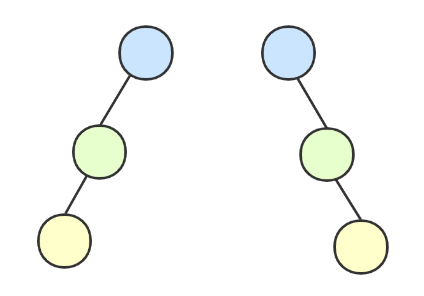### 满二叉树¶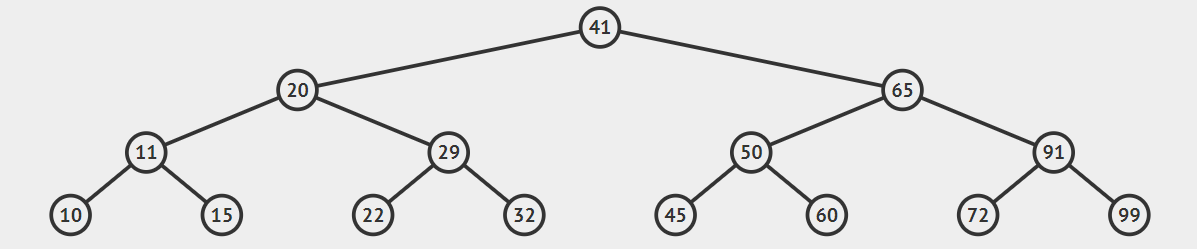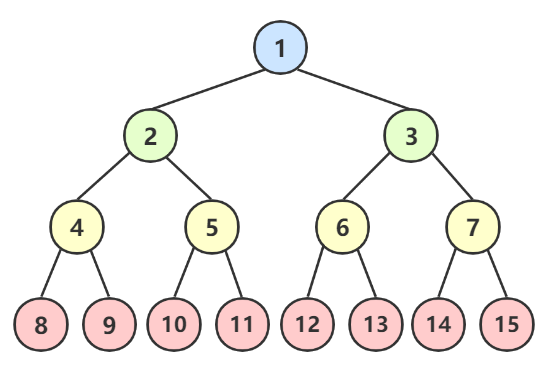1. 叶结点只能出现在最下层，其他层出现就不可能达成平衡
2. 分支结点的度必须 = 2，否则就是缺胳膊少腿了。
3. 在同样深度的二叉树中，满二叉树的结点树最多，叶子最多。

### 完全二叉树¶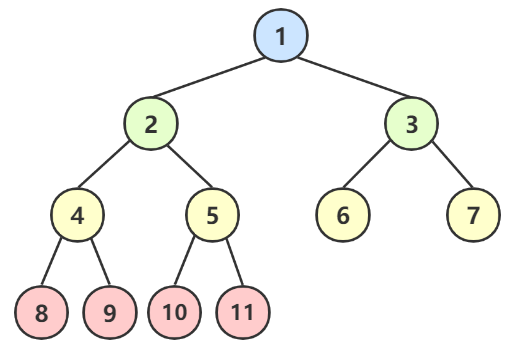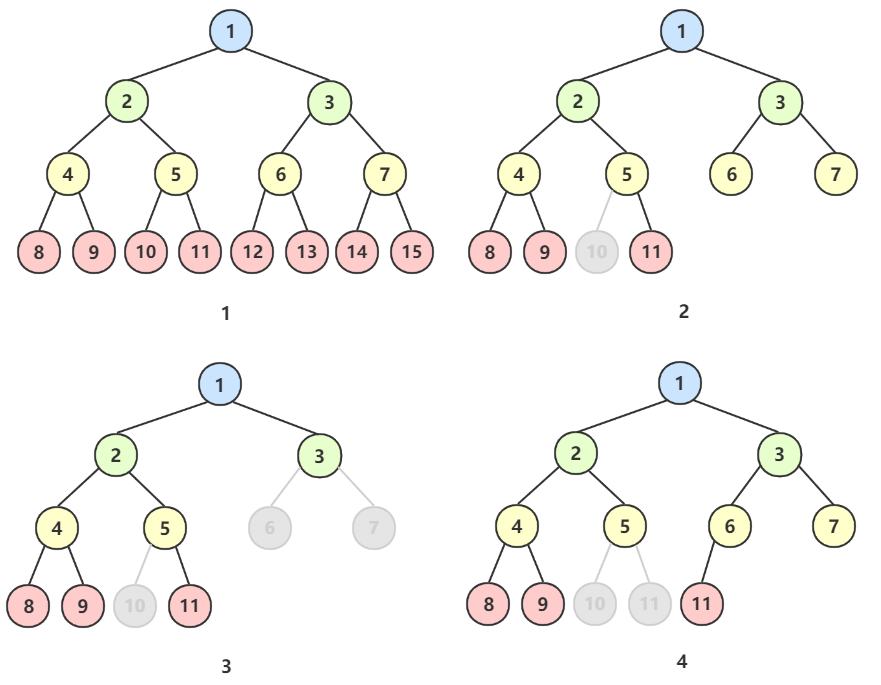1. 叶子结点只能出现在最下面两层。
2. 最下层的叶子一定集中在左部连续位置。
3. 倒数两层，若有叶子结点，一定都在右部连续位置
4. 如果结点度为 1，则该结点只有左孩子，不能只有右孩子。
5. 同样结点数的二叉树，完全二叉树的深度最小。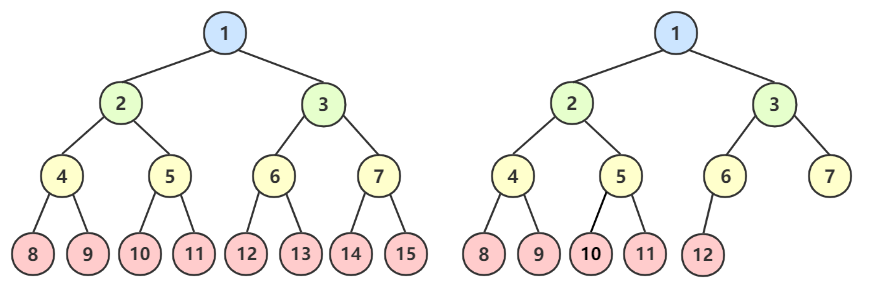### 性质¶Note

Tip

• 如果 i = 1，则结点 i 是二叉树的根，无双亲；
• 如果 i > 1，则其双亲结点是第 $$⌊i/2⌋$$ 个；
• 如果 2i > n，则结点 i 无左孩子（结点 i 为叶子结点）；否则其左孩子是结点 2i；
• 如果 2i+1 > n，则结点 i 无右孩子；否则其右孩子是结点 2i+1。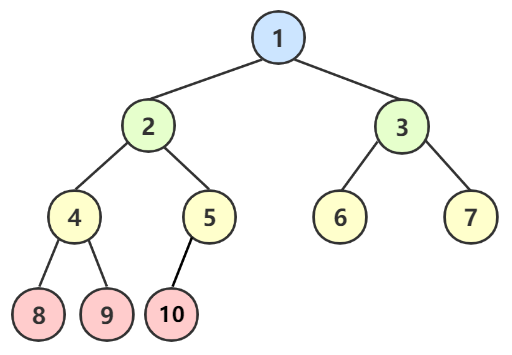• 如果 i = 1，根节点。
• 如果 i = 7，双亲就是 $$⌊7/2⌋ = ⌊3.5⌋ = 3$$
• 如果 i = 6，(2i = 2*6 = 12) > (10 = n)，所以6无左孩子；
• 如果 i = 4，(2i + 1 = 9) > 10，所以4的右孩子是9。

• i = 1，为根结点
• i 的双亲是 ⌊i/2⌋
• i 的左孩子是 2i
• i 的右孩子是 2i + 1

### 存储结构¶

typedef struct {
int id;
char* name;
} Elem;

typedef struct BTNode{
Elem data;            // 数据域
struct BTNode* lchild; // 左孩子
struct BTNode* rchild; // 右孩子
} BTNode;

typedef struct {
BTNode* root; // 根结点
int depth;    // 树的度
int count;    // 总结点数
} BTree;


### 遍历¶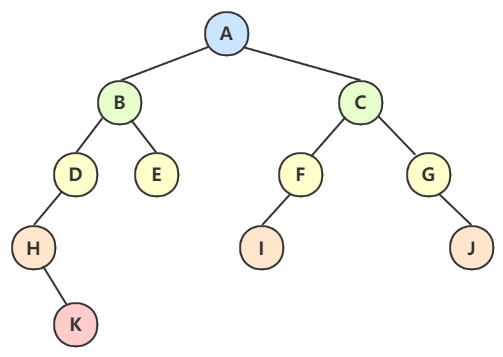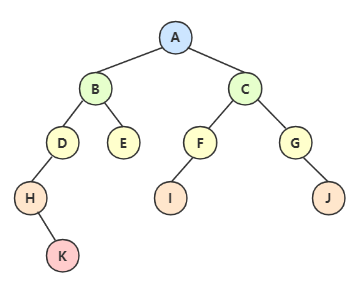void PreOrderTraverse(BTNode T) {
if (T == NULL) return;
printf("%c", T->data);
PreOrderTraverse(T->lchild);
PreOrderTraverse(T->rchild);
}void InOrderTraverse(BTNode T) {
if (T == NULL) return;
InOrderTraverse(T->lchild);
printf("%c", T->data);
InOrderTraverse(T->rchild);
}void PostOrderTraverse(BTNode T) {
if (T == NULL) return;
PostOrderTraverse(T->lchild);
PostOrderTraverse(T->rchild);
printf("%c", T->data);
}void SequenceTraverse(BTree T) {
EnQueue(T->root);
BTNode* root = DeQueue()
for (int i = 0; i < T->count; i++) {
if (root) printf("%c", root->data);
if (root->lchild) EnQueue(root->lchild);
if (root->rchild) EnQueue(root->rchild);
}
}


#### 总结¶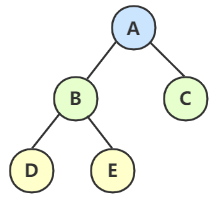• 前序 DLR：ABDEC
• 中序 LDR：DBEAC
• 后序 LRD：DEBCA
• 层次遍历：ABCDE

#### 例题¶

1. 前序的第一位或后序的最后一位，一定是根节点，所以这题的根结点就是 A。
2. 接着看 A 在中序序列的位置，可以看出 CB 是 A 的左孩子，EDF是 A 的右孩子；然后在前序中就可以确定 BC 为一组，DEF 为一组。

3. 二叉树的子树也是二叉树。根据这个道理，从前序中可以知道 B是左子树的根结点，同理D是右子树的根结点。

4. 拿着 B 和 D 到中序序列中分析，分出左右子树，以此类推。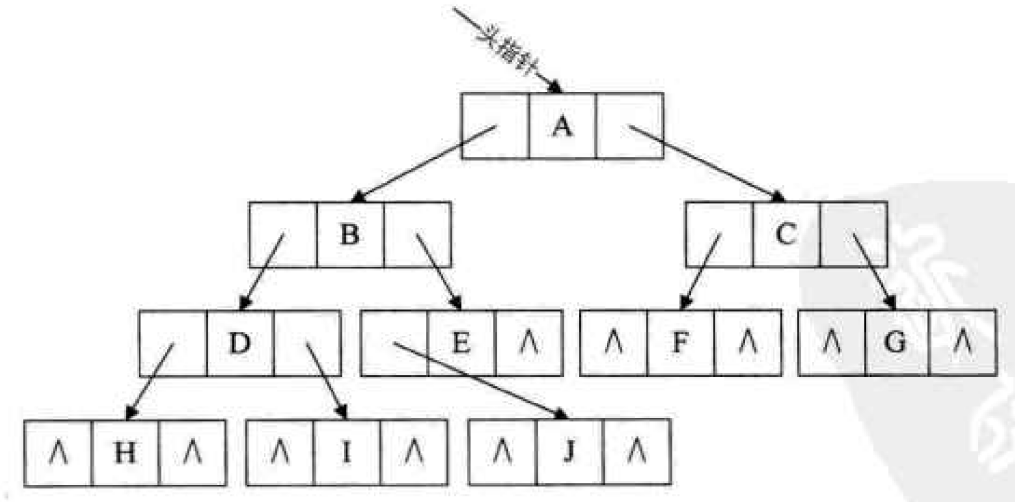• 如果有左子树，则左指针域指向左子树 如果没有左子树，则左指针指向 遍历前驱元素
• 如果有右子树，则右指针域指向右子树 如果没有右子树，则右指针指向 遍历后继元素

### 实现细节¶

typedef struct BTNode{
Elem data;             // 数据域
struct BTNode* lchild; // 左孩子
struct BTNode* rchild; // 右孩子
bool ltag;
bool rtag;
} BTNode;


TTNode* pre;     // 全局变量，指向刚刚访问过的结点
if(T) {

/** 线索化 begin */
if(!T->lchild) {    // 没有左孩子
T->ltag = false;        // 线索标志
T->lchild = pre;    // 指向前驱元素
}
if(!pre->rchild) {    // 前驱结点没有右孩子
pre->rtag = false;      // 线索标志
pre->rchild = T;    // 前驱右指针指向当前指针
}
pre = T;    // 保持 pre 指向 T 的前驱
/** 线索化 end */

}
}


## 转换¶

### 树转二叉树¶

1. 连接：兄弟之间连线
2. 断开：双亲结点只保留最左孩子，其余都断开
3. 旋转：调整好位置
4. 保留的做左子树，连接的做右子树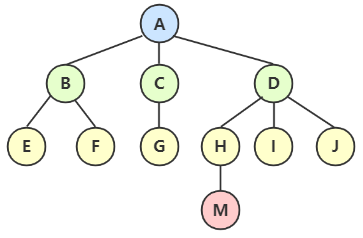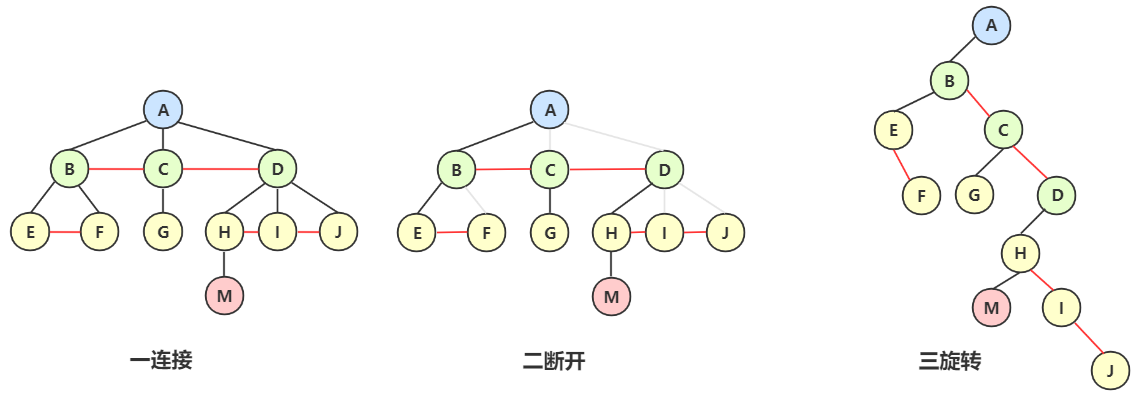• 第一步：兄弟结点之间连接起来。例如 BCD 之间都连接起来了。
• 第二步：除了双亲跟左孩子，其他联系都断开。例如A只保留与 B，断开与 CD 的关系。
• 第三步：旋转调整。保留下来的作为左子树，连接得来的作为右子树。例如 B 是保留下来的，作为左子树，CD 是连接出来的，作为右子树。

Tip

### 二叉树转树¶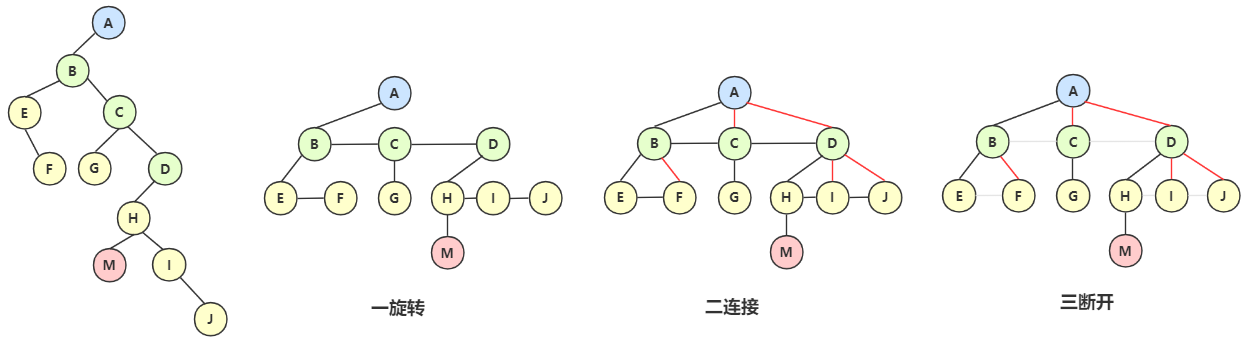• 第一步：旋转，将右子树都旋转都同一层
• 第二步：连接右子树结点与双亲结点
• 第三步：断开兄弟间的连线

### 二叉树转森林¶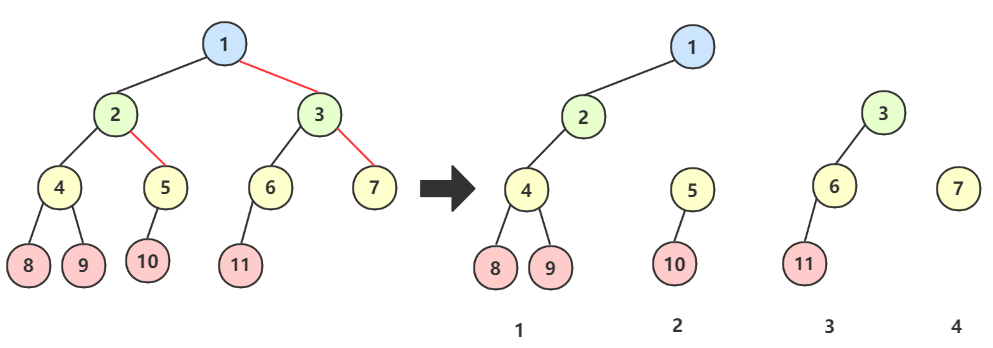## 哈夫曼树¶

• 路径：两个结点之间那根线就叫路径
• ：路径上的数字。
• 带权路径：两个结点之间那根线带数字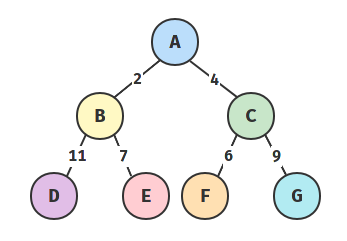• 路径长度：从树中一个结点到另一个结点之间的分支构成这两个结点之间的路径，路径上的分支数目称作路径长度
• 树的路径长度：从树根到每一个结点的路径长度之和
• 树的带权路径长度：树中所有叶子结点的带权路径长度之和

通常记作 $$WPL=\sum^n_{k=1}{w_kl_k}$$。（$$l$$ 为结点 $$k$$ 的层数，从 0 开始计算）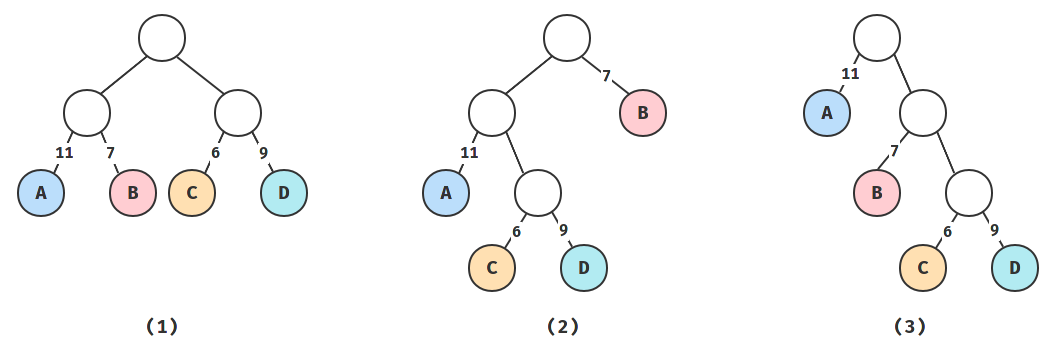1. $$WPL = 11 \times 2 + 7 \times 2 + 6 \times 2 + 9 \times 2 = 66$$
2. $$WPL = 11 \times 2 + 7 \times 1 + 6 \times 3 + 9 \times 3 = 74$$
3. $$WPL = 11 \times 1 + 7 \times 2 + 6 \times 3 + 9 \times 3 = 70$$

### 哈夫曼编码¶

a b c d e f
001 010 011 100 101 110

aaabbaacdeefdeaeff 编码后为 001001001010010001001011100101101110100101001101110110。18 个字符共编成 54 个二进制位。

### 编码步骤¶

1. 统计各种字符的出现次数构成一个序列
2. 对序列进行排序
3. 选取 2 个最小的作为叶子结点，父结点为两次数之和
4. 将选出的两个数从序列中删除，将父结点加入序列
5. 重复步骤 2，直到序列为空。

• 保持任何左侧结点都比右侧的兄弟结点小/大。
• 构造完成以后，给每一条路径填充权值 0 或 1。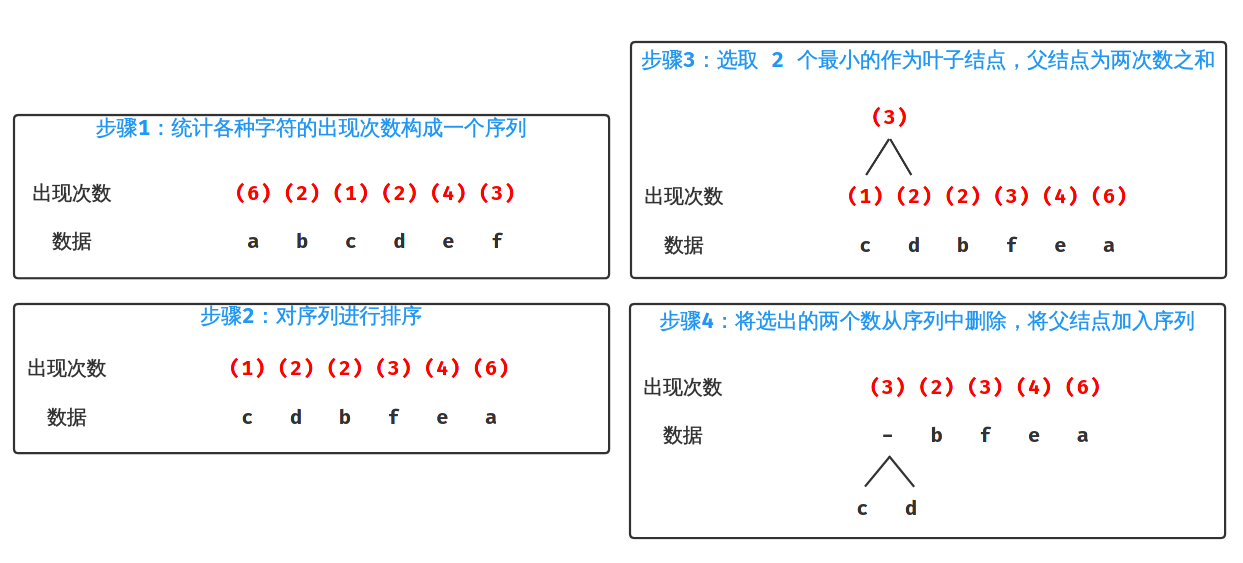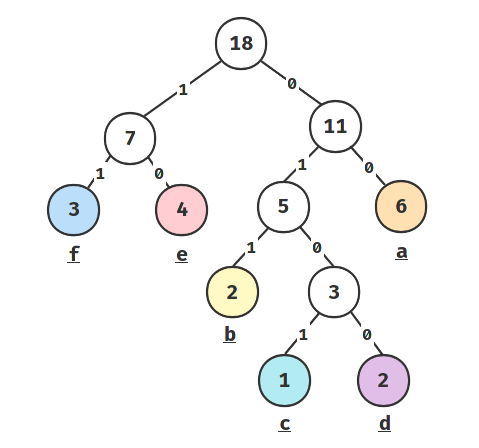a b c d e f
00 011 0101 0100 10 11

### 代码实现¶

while (序列长度 >= 1) {
将序列排序
栈顶 2 个元素出栈
构造子树
将父结点的值入栈
}


// DataElement.h

#ifndef DATAELEMENT_H_INCLUDED
#define DATAELEMENT_H_INCLUDED

#include <stdio.h>
#include <stdlib.h>

#define MAXSIZE 10

typedef struct Node {
char data;
int value;
struct Node* lNode;
struct Node* rNode;
int lWeight;
int rWeight;
} Node;

typedef struct Stack {
Node* datas[MAXSIZE];
int top;
} Stack;

typedef struct {
char data;
int times;
} seq;

#endif

// Huffman.h

#ifndef HUFFMAN_H_INCLUDED
#define HUFFMAN_H_INCLUDED

#include <stdbool.h>
#include "DataElement.h"
#define len(x) sizeof(x)/sizeof(x)

// 将序列排序
bool sort(Stack*);

// 出栈两个元素
void pop(Stack* s, Node* nodeArr);

// 构造子树
Node* buildSubTree(Node* nodeArr);

// 父结点入栈
void push(Stack* s, Node* parent);

bool initStack(Stack* s, seq arr[], int arrLen);
void printStack(Stack* s);
void frontPrint(Node* root);
void midPrint(Node* root);
void stuffWeight(Node* root);

#endif

// Huffman.c

#include "Huffman.h"

// 将序列排序
/**
* @name 直接插入排序算法
* 循环数组长度 -1 次，每次循环将当前目标数与前面部分（已排好序）比较
* 前一个数大于当前数则交换位置，一直比较一直换，直到前面的数小于当前数时，退出比较，换下一个目标数继续比较。
*/
bool sort(Stack* s)
{
int i;
for (i = 1; i < s->top + 1; i++) { // 一共循环数组长度 -1 次
int j;
for (j = i; j > 0; j--) {
Node* prev = s->datas[i - 1];
Node* curr = s->datas[i];
if (prev->value < curr->value) { // 若前一个小于后一个，则交换两个结点，当前目标数位置-1
Node* tmp = s->datas[i - 1];
s->datas[i - 1] = s->datas[i];
s->datas[i] = tmp;
tmp = NULL;
i--;
} else {
break;
}
}
}
}

// 出栈两个元素
void pop(Stack* s, Node* nodeArr)
{
nodeArr = s->datas[s->top--];
nodeArr = s->datas[s->top--];
}

// 构造子树
Node* buildSubTree(Node* nodeArr)
{
Node* parent = (Node*)malloc(sizeof(Node));
parent->data = '-';
parent->value = nodeArr->value + nodeArr->value;
parent->lNode = nodeArr;
parent->rNode = nodeArr;
parent->lWeight = parent->rWeight = -1;
return parent;
}

// 父结点入栈
void push(Stack* s, Node* parent)
{
s->datas[++s->top] = parent;
}

// 填充每条路径上的权值
void stuffWeight(Node* root)
{
if (root) {
if (root->lNode)
root->lWeight = 1;
if (root->rNode)
root->rWeight = 0;
stuffWeight(root->lNode);
stuffWeight(root->rNode);
}
}

// 初始化栈
bool initStack(Stack* s, seq arr[], int arrLen)
{
int i;
for (i = 0; i < MAXSIZE; i++)
s->datas[i] = NULL;

s->top = -1;

// 将数组填充进栈里
for (i = 0; i < arrLen; i++) {
Node* node = (Node*)malloc(sizeof(Node));
node->data = arr[i].data;
node->value = arr[i].times;
node->lNode = node->rNode = NULL;
node->lWeight = node->rWeight = -1;
push(s, node);
}
return true;
}

// 前序遍历打印
void frontPrint(Node* root)
{
if (root != NULL) {
printf("%c %2d; %2d, %2d\n", root->data, root->value, root->lWeight, root->rWeight);
frontPrint(root->lNode);
frontPrint(root->rNode);
}
}

// 中序遍历打印
void midPrint(Node* root)
{
if (root != NULL) {
midPrint(root->lNode);
printf("%c %2d; %2d, %2d\n", root->data, root->value, root->lWeight, root->rWeight);
midPrint(root->rNode);
}
}

// main.c

#include "Huffman.c"
#include "tools.c"

void BuildHuffman()
{

seq arr[] = { { 'a', 6 }, { 'b', 2 }, { 'c', 1 }, { 'd', 2 }, { 'e', 4 }, { 'f', 3 } };
Stack s;
initStack(&s, arr, len(arr));
Node* popArr;

while (s.top >= 1) {
sort(&s);
pop(&s, popArr);
Node* parent = buildSubTree(popArr);
push(&s, parent);
}
stuffWeight(s.datas[s.top]);
printStack(&s);
frontPrint(s.datas[s.top]);
printf("\n");
midPrint(s.datas[s.top]);
}

void main()
{
BuildHuffman();
}